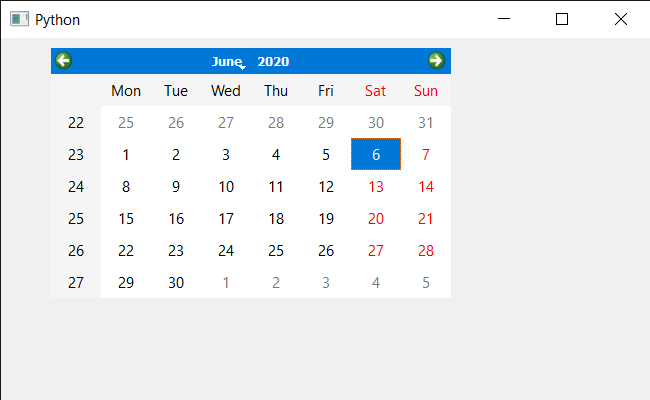GeeksforGeeks App
Open AppBrowser
Continue

# PyQt5 QCalendarWidget – Setting Property

In this article we will see how we can set properties information to the QCalendarWidget, properties are the information added by the developer to tell about the properties of the calendar, for example if calendar has a blocked future date, developer will add this property so that other developer can see this and get to know about the property.

In order to do this we will use `setProperty` method with the QCalendarWidget object.

Syntax : calendar.setProperty(property, value)

Argument : It takes two argument one is string and other is integer

Return : It return None

Below is the implementation

## Python3

 `# importing libraries``from` `PyQt5.QtWidgets ``import` `*` `from` `PyQt5 ``import` `QtCore, QtGui``from` `PyQt5.QtGui ``import` `*` `from` `PyQt5.QtCore ``import` `*` `import` `sys`` ` ` ` `class` `Window(QMainWindow):`` ` `    ``def` `__init__(``self``):``        ``super``().__init__()`` ` `        ``# setting title``        ``self``.setWindowTitle(``"Python "``)`` ` `        ``# setting geometry``        ``self``.setGeometry(``100``, ``100``, ``650``, ``400``)`` ` `        ``# calling method``        ``self``.UiComponents()`` ` `        ``# showing all the widgets``        ``self``.show()`` ` `    ``# method for components``    ``def` `UiComponents(``self``):`` ` `        ``# creating a QCalendarWidget object``        ``self``.calendar ``=` `QCalendarWidget(``self``)`` ` `        ``# setting geometry to the calendar``        ``self``.calendar.setGeometry(``50``, ``10``, ``400``, ``250``)`` ` `        ``# setting cursor``        ``self``.calendar.setCursor(Qt.PointingHandCursor)`` ` `        ``# setting properties``        ``self``.calendar.setProperty(``"Blocked Dates ?: "``, ``0``)`` ` ` ` ` ` `# create pyqt5 app``App ``=` `QApplication(sys.argv)`` ` `# create the instance of our Window``window ``=` `Window()``window.calendar.destroy()`` ` ` ` `# start the app``sys.exit(App.``exec``())`

Output :My Personal Notes arrow_drop_up